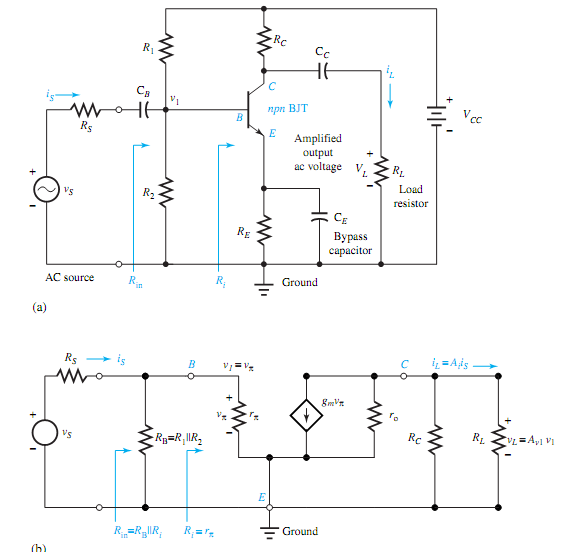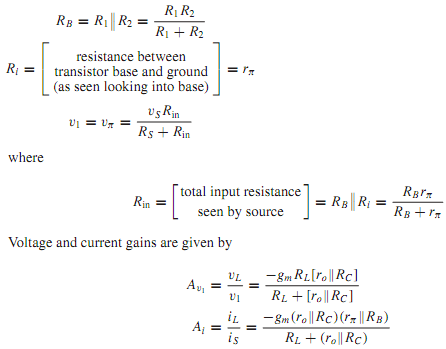Common-emitter configuration, Electrical Engineering

Assignment Help:

Q. Common-Emitter Configuration?

The emitter part of a circuit being common to both the input and the output portions, Figure (a) illustrates a common-emitter (CE) BJT amplifier. The resistors R1, R2, RC, and RE are primarily set by biasing. The input ac source is represented by its Thévenin equivalent. The amplified output ac voltage vL appears across the load resistor RL, which could represent the input resistance of the next stage in a cascade. Capacitors CB,CC, and CE are so chosen that they represent short circuits at the lowest frequency of interest. CE would be made large enough so that 1/ωCE is small relative to RE in parallel with the impedance looking into the emitter at the smallest ω of interest.Similarly, the reactances of CC and CB would be chosen small relative to the resistances in their parts of the circuit. Capacitors CB and CC appear as short circuits to the ac signals, but block the dc voltages and currents out of one part of the circuit from coupling with  another part. Capacitor CE, known as the bypass capacitor, bypasses the ac current around RE so that no significant ac voltage is generated  across RE, and helps to increase the gain.

The small-signal ac equivalent circuit is shown in Figure (b), in which the small- signal model of Figure for the transistor is used. While omitting the details of analysis and summarizing the results, we haveImpact of diversity, Both GSM and DECT use GMSK, but with different Gaussia...

Both GSM and DECT use GMSK, but with different Gaussian filters (BGT = 0.3 in GSM, BGT = 0.5 in DECT). What are the advantages of having a larger bandwidth time product? Why is the

What is the maximum entrance angle max, Calculate the N.A of a step index f...

Calculate the N.A of a step index fibre having n 1 =1.48 and n 2 =1.46. What is the maximum entrance angle ψ max for this fibre if the outer medium is air with n 1 =1.0? Given

Governer, governer characteristics

governer characteristics

Explain the properties and application of glass, Explain the properties and...

Explain the properties and application of glass. Glass: Ordinary glass is a fine insulator but is too brittle to be used for anything although scientific instrument parts, accu

Calculate k for n-channel enhancement mosfet, Q. An n-channel enhancement M...

Q. An n-channel enhancement MOSFET operates in the active region with very large V A , v GS = 6V, V = 4 V, and i = 1 mA. Calculate K.

Effect of frequency - stepper motor , Effect of Frequency a.From equa...

Effect of Frequency a.From equation for given  H p and μ P v α √f b.From  equation the depth of penetration for a given  material δ α 1/ √f c.From  equation hyst

Address Bus The address  bus is the group  of 16 lines. It is used  to carry  the address of memory  location and  addresses  of input  and output  ports as shown. It is a unid

Evaluate maximum directive gain, Q. For a pyramidal-horn antenna, the maxim...

Q. For a pyramidal-horn antenna, the maximum directive gain is given by occurring when the aperture dimensions are A ∼ = √3λLandB = 0.81A. The principal-plane beam- widths

Write a note on clampers, Q. Write a note on clampers ? Clamper is a ci...

Q. Write a note on clampers ? Clamper is a circuit that "clamps" a signal to a different dc level. The different types of clampers are positive negative and biased clampers.

Explain how materials can be classified in three groups, Explain how materi...

Explain how materials can be classified into three groups on the basis of atomic structure. An atom has a positively charged nucleus surrounded through a group of negatively ch